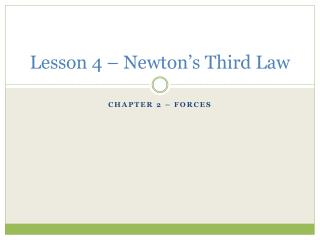DownloadDownload PresentationLesson 4 – Newton’s Third Law

# Lesson 4 – Newton’s Third Law

Télécharger la présentation## Lesson 4 – Newton’s Third Law

- - - - - - - - - - - - - - - - - - - - - - - - - - - E N D - - - - - - - - - - - - - - - - - - - - - - - - - - -

1. ### Lesson 4 – Newton’s Third Law

Chapter 2 – Forces
2. Newton’s Third Law Newton’s third law of motion states that if one object exerts a force on another object, then the second object exerts a force of equal strength in the opposite direction on the first object. Another way to state Newton’s third law is that for every action there is an equal but opposite reaction.
3. Action – Reaction Pairs Action- reaction pairs explain how a gymnast can flip over a vaulting horse, how a kayaker can move through water, and how a dog can leap off the ground. The action and reaction forces do not cancel each other out because they are acting on different objects.
4. Momentum All moving objects have what Newton called a “ quantity of motion.” Momentum is a characteristic of a moving object that is related to the mass and the velocity of the object. The momentum of a moving object can be determined by multiplying the object’s mass and velocity. Momentum = Mass x Velocity
5. The more momentum a moving object has, the harder it is to stop. The mass of an object affects the amount of momentum the object has. For example: you can catch a baseball moving at 20 m/s, but you cannot stop a car moving at the same speed. The car has more momentum because it has a greater mass.
6. Conservation of Momentum The law of conservation of momentum states that, in the absence of outside forces, the total momentum of objects that interact does not change. The amount of momentum is the same before and after they interact. The total momentum of any group of objects remains the same, or is conserved, unless outside forces act on the objects. Friction is an example of an outside force.